# Styling Visual Attributes¶

## Using Palettes¶

Palettes are sequences (lists or tuples) of RGB(A) hex strings that define a colormap and be can set as the `color` attribute of many plot objects from `bokeh.plotting`. Bokeh offers many of the standard Brewer palettes, which can be imported from the `bokeh.palettes` module. For example, importing “Spectral6” gives a six element list of RGB(A) hex strings from the Brewer “Spectral” colormap.

```>>> from bokeh.palettes import Spectral6
>>> Spectral6
['#3288bd', '#99d594', '#e6f598', '#fee08b', '#fc8d59', '#d53e4f']
```

All of the standard palettes included in bokeh can be found at bokeh.palettes. Custom palettes can be made by creating sequences of RGB(A) hex strings.

## Using Mappers¶

Color Mappers allow you to encode some data sequence into a palette of colors based on the value in that sequence. The mappers are then set as the `color` attribute on marker objects. Bokeh includes several types of mappers to encode colors:

• `bokeh.transform.factor_cmap`: Maps colors to specific categorical elements see Handling Categorical Data for more detail.

• `bokeh.transform.linear_cmap`: Maps a range of numeric values across the available colors from high to low. For example, a range of [0,99] given the colors [‘red’, ‘green’, ‘blue’] would be mapped as follows:

```    x < 0  : 'red'     # values < low are clamped
0 >= x < 33 : 'red'
33 >= x < 66 : 'green'
66 >= x < 99 : 'blue'
99 >= x      : 'blue'    # values > high are clamped
```
• `bokeh.transform.log_cmap`: Similar to `linear_cmap` but uses a natual log scale to map the colors.

These mapper functions return a `DataSpec` property that can be passed to the color attribute of the glyph. The returned dataspec includes a `bokeh.transform` which can be accessed to use the mapper in another context such as to create a `ColorBar` as in the example below:

```from bokeh.plotting import figure, show, output_file
from bokeh.models import ColumnDataSource, ColorBar
from bokeh.palettes import Spectral6
from bokeh.transform import linear_cmap

output_file("styling_linear_mappers.html", title="styling_linear_mappers.py example")

x = [1,2,3,4,5,7,8,9,10]
y = [1,2,3,4,5,7,8,9,10]

#Use the field name of the column source
mapper = linear_cmap(field_name='y', palette=Spectral6 ,low=min(y) ,high=max(y))

source = ColumnDataSource(dict(x=x,y=y))

p = figure(plot_width=300, plot_height=300, title="Linear Color Map Based on Y")

p.circle(x='x', y='y', line_color=mapper,color=mapper, fill_alpha=1, size=12, source=source)

color_bar = ColorBar(color_mapper=mapper['transform'], width=8,  location=(0,0))

show(p)
```

## Visual Properties¶

In order to style the visual attributes of Bokeh plots, you first must know what the available properties are. The full Reference Guide will list all the properties of every object individually, though there are three broad groups of properties that show up often. They are:

• line properties line color, width, etc.

• fill properties fill color, alpha, etc.

• text properties font styles, colors, etc.

Below is more detail about each of these.

### Line Properties¶

`line_color`

color to use to stroke lines with

`line_width`

line stroke width in units of pixels

`line_alpha`

floating point between 0 (transparent) and 1 (opaque)

`line_join`

how path segments should be joined together

`line_cap`

how path segments should be terminated

`line_dash`

a line style to use

• `'solid'`

• `'dashed'`

• `'dotted'`

• `'dotdash'`

• `'dashdot'`

• an array of integer pixel distances that describe the on-off pattern of dashing to use

• a string of spaced integers matching the regular expression ‘^(\d+(\s+\d+)*)?\$’ that describe the on-off pattern of dashing to use

`line_dash_offset`

the distance in pixels into the `line_dash` that the pattern should start from

### Fill Properties¶

`fill_color`

color to use to fill paths with

`fill_alpha`

floating point between 0 (transparent) and 1 (opaque)

### Hatch Properties¶

`hatch_color`

color to use to stroke hatch patterns with

`hatch_alpha`

floating point between 0 (transparent) and 1 (opaque)

`hatch_weight`

line stroke width in units of pixels

`hatch_scale`

a rough measure of the “size” of a pattern. This value has different specific meanings, depending on the pattern.

`hatch_pattern`

a string name (or abbreviation) for a built-in pattern, or a string name of a pattern provided in `hatch_extra`. The built-in patterns are:

Built in Hatch Patterns

FullName

Abbreviation

Example

`blank`

`" "``dot`

`"."``ring`

`"o"``horizontal_line`

`"-"`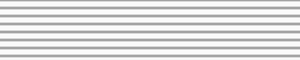`vertical_line`

`"|"`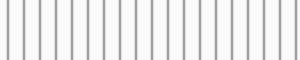`cross`

`"+"`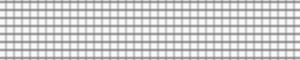`horizontal_dash`

`'"'`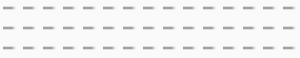`vertical_dash`

`":"`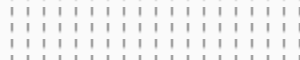`spiral`

`"@"`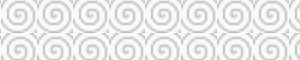`right_diagonal_line`

`"/"`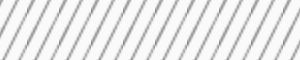`left_diagonal_line`

`"\\"`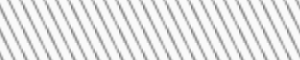`diagonal_cross`

`"x"`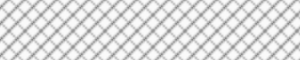`right_diagonal_dash`

`","``left_diagonal_dash`

`"`"`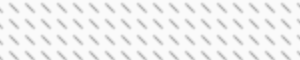`horizontal_wave`

`"v"``vertical_wave`

`">"``criss_cross`

`"*"`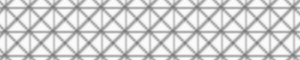`hatch_extra`

a dict mapping string names to custom pattern implementations. The name can be referred to by `hatch_pattern`. For example, if the follwing value is set for `hatch_extra`:

```hatch_extra={ 'mycustom': ImageURLTexture(url=...) }
```

then the name `"mycustom"` may be set as a `hatch_pattern`.

### Text Properties¶

`text_font`

font name, e.g., `'times'`, `'helvetica'`

`text_font_size`

font size in px, em, or pt, e.g., `'12pt'`, `'1.5em'`

`text_font_style`

font style to use

• `'normal'` normal text

• `'italic'` italic text

• `'bold'` bold text

`text_color`

color to use to render text with

`text_alpha`

floating point between 0 (transparent) and 1 (opaque)

`text_align`

horizontal anchor point for text: `'left'`, `'right'`, `'center'`

`text_baseline`

vertical anchor point for text

• `'top'`

• `'middle'`

• `'bottom'`

• `'alphabetic'`

• `'hanging'`

Note

There is currently only support for filling text. An interface to stroke the outlines of text has not yet been exposed.

### Visible property¶

Glyph renderers, axes, grids, and annotations all have a visible property that can be used to turn them on and off.

```from bokeh.io import output_file, show
from bokeh.plotting import figure

# We set-up a standard figure with two lines
p = figure(plot_width=500, plot_height=200, tools='')
visible_line = p.line([1, 2, 3], [1, 2, 1], line_color="blue")
invisible_line = p.line([1, 2, 3], [2, 1, 2], line_color="pink")

# We hide the xaxis, the xgrid lines, and the pink line
invisible_line.visible = False
p.xaxis.visible = False
p.xgrid.visible = False

output_file("styling_visible_property.html")

show(p)
```

This can be particularly useful in interactive examples with bokeh server or CustomJS.

```from bokeh.io import output_file, show
from bokeh.plotting import figure
from bokeh.layouts import layout
from bokeh.models import Toggle, BoxAnnotation

output_file("styling_visible_annotation_with_interaction.html")

p = figure(plot_width=600, plot_height=200, tools='')
p.line([1, 2, 3], [1, 2, 1], line_color="blue")
pink_line = p.line([1, 2, 3], [2, 1, 2], line_color="pink")

green_box = BoxAnnotation(left=1.5, right=2.5, fill_color='green', fill_alpha=0.1)

# Use js_link to connect button active property to glyph visble property

toggle1 = Toggle(label="Green Box", button_type="success", active=True)

toggle2 = Toggle(label="Pink Line", button_type="success", active=True)

show(layout([p], [toggle1, toggle2]))
```

### Specifying Colors¶

Colors properties are used in many places in Bokeh, to specify the colors to use for lines, fills or text. Color values can be provided in any of the following ways:

• any of the 147 named CSS colors, e.g `'green'`, `'indigo'`

• an RGB(A) hex value, e.g., `'#FF0000'`, `'#44444444'`

• a 3-tuple of integers (r,g,b) between 0 and 255

• a 4-tuple of (r,g,b,a) where r, g, b are integers between 0 and 255 and a is a floating point value between 0 and 1

Warning

Supplying lists of RGB or RGBA color tuples as color arguments (either directly or as a DataSource column reference) doesn’t work. You may read a discussion of the issue on our project GitHub page. Suggested work-arounds include using lists of:

• RGB hexadecimal values

• bokeh.colors.RGB objects (i.e. `[RGB(255, 0, 0), RGB(0, 255, 0)"]`)

• CSS-format RGB/RGBA strings (i.e. `["rgb(255, 0, 0)", "rgb(0, 255, 0)"]`)

Color alpha can be specified in multiple ways for the visual properties. This can be by specifying the alpha directly with `line|fill_alpha`, or by providing the alpha through the RGBA 4-tuple for the `line|fill_color`.

Additionally, there is also the freedom to use a combination of the two, or no alpha at all. The following figure demonstrates each possible combination of the inputs for line and fill alphas:

Note

If using the bokeh.plotting interface, another option is to specify `color` and/or `alpha` as a keyword, as well as the demonstrated color properties. These inputs work by applying the provided value to both of the corresponding `line` and `fill` properties. However, you can still provide `fill|line_alpha` or `fill|line_color` in combination with the `color`/`alpha` keywords, and the former will take precedence.

### Styling Arrow Annotations¶

There are several ArrowHead subtypes that can be applied to Arrow annotations. Setting the start or end property to None will cause no arrow head to be applied at the specified arrow end. Double-sided arrows can be created by setting both start and end styles. Setting visible to false on an arrow will also make the corresponding arrow head invisible.

### Screen Units and Data-space Units¶

Screen units use raw numbers of pixels to specify height or width, while data-space units are relative to the data and the axes of the plot. For example, in a 400 pixel by 400 pixel graph with x and y axes ranging from 0 through 10, a glyph one fifth as wide and tall as the graph would be 80 screen units or 2 data-space units.

## Selecting Plot Objects¶

As described in Defining Key Concepts, Bokeh plots comprise graphs of objects that represent all the different parts of the plot: grids, axes, glyphs, etc. In order to style Bokeh plots, it is necessary to first find the right object, then set its various attributes. Some objects have convenience methods to help find the objects of interest (see Axes, Grids, and Legends). But there is also a `select()` method on `Plot` that can be used to query for Bokeh plot objects more generally.

For example, you can query for objects by type. The following snippet returns all the PanTool objects a plot has:

```>>> p.select(type=PanTool)
[<bokeh.models.tools.PanTool at 0x106608b90>]
```

The `select()` method can query on other attributes as well:

```>>> p.circle(0, 0, name="mycircle")
<bokeh.plotting.Figure at 0x106608810>

>>> p.select(name="mycircle")
[<bokeh.models.renderers.GlyphRenderer at 0x106a4c810>]
```

This sort of query can be especially useful for styling visual attributes of Glyphs.

## Plots¶

`Plot` objects themselves have many visual characteristics that can be styled: the dimensions of the plot, backgrounds, borders, outlines, etc. This section describes how to change these attributes of a Bokeh plot. The example code primarily uses the bokeh.plotting interface to create plots, however the instructions apply regardless of how a Bokeh plot was created.

### Dimensions¶

The dimensions (width and height) of a `Plot` are controlled by `plot_width` and `plot_height` attributes. These values are in screen units, and they control the size of the entire canvas area, including any axes or titles (but not the toolbar). If you are using the bokeh.plotting interface, then these values can be passed to `figure()` as a convenience:

```from bokeh.plotting import figure, output_file, show

output_file("dimensions.html")

# create a new plot with specific dimensions
p = figure(plot_width=700)
p.plot_height = 300

p.circle([1, 2, 3, 4, 5], [2, 5, 8, 2, 7], size=10)

show(p)
```

### Responsive Dimensions¶

For control over how the plot scales to fill its container, see the documentation for bokeh.models.layouts, in particular the `sizing_mode` property of `LayoutDOM`.

If you set `sizing_mode`, the `plot_width` and `plot_height` may immediately change when a plot is rendered to fill the container. However, those parameters will be used to calculate the initial aspect ratio for your plot, so you may want to keep them. Plots will only resize down to a minimum of 100px (height or width) to prevent problems in displaying your plot.

### Title¶

The styling of the plot title is controlled by the properties of `Title` annotation, which is available as the `.title` property on the `Plot`. Most of the standard Text Properties are available, with the exception of `text_align` and `text_baseline` which do not apply. For positioning the title relative to the entire plot, use the properties `align` and `offset`.

As an example, to set the color and font style of the title text, use `plot.title.text_color`:

```from bokeh.plotting import figure, output_file, show

output_file("title.html")

p = figure(plot_width=400, plot_height=400, title="Some Title")
p.title.text_color = "olive"
p.title.text_font = "times"
p.title.text_font_style = "italic"

p.circle([1, 2, 3, 4, 5], [2, 5, 8, 2, 7], size=10)

show(p)
```

### Background¶

The background fill style is controlled by the `background_fill_color` and `background_fill_alpha` properties of the `Plot` object:

```from bokeh.plotting import figure, output_file, show

output_file("background.html")

p = figure(plot_width=400, plot_height=400)
p.background_fill_color = "beige"
p.background_fill_alpha = 0.5

p.circle([1, 2, 3, 4, 5], [2, 5, 8, 2, 7], size=10)

show(p)
```

### Border¶

The border fill style is controlled by the `border_fill_color` and `border_fill_alpha` properties of the `Plot` object. You can also set the minimum border on each side (in screen units) with the properties

`min_border_left`

`min_border_right`

`min_border_top`

`min_border_bottom`

Additionally, setting `min_border` will apply a minimum border setting to all sides as a convenience. The `min_border` default value is 40px.

```from bokeh.plotting import figure, output_file, show

output_file("border.html")

p = figure(plot_width=400, plot_height=400)
p.border_fill_color = "whitesmoke"
p.min_border_left = 80

p.circle([1,2,3,4,5], [2,5,8,2,7], size=10)

show(p)
```

### Outline¶

The styling of the outline of the plotting area is controlled by a set of Line Properties on the `Plot`, that are prefixed with `outline_`. For instance, to set the color of the outline, use `outline_line_color`:

```from bokeh.plotting import figure, output_file, show

output_file("outline.html")

p = figure(plot_width=400, plot_height=400)
p.outline_line_width = 7
p.outline_line_alpha = 0.3
p.outline_line_color = "navy"

p.circle([1,2,3,4,5], [2,5,8,2,7], size=10)

show(p)
```

## Glyphs¶

To style the fill, line, or text properties of a glyph, it is first necessary to obtain a specific `GlyphRenderer`. When using the bokeh.plotting interface, the glyph functions return the renderer:

```>>> r = p.circle([1,2,3,4,5], [2,5,8,2,7])
>>> r
<bokeh.models.renderers.GlyphRenderer at 0x106a4c810>
```

Then, the glyph itself is obtained from the `.glyph` attribute of a `GlyphRenderer`:

```>>> r.glyph
<bokeh.models.markers.Circle at 0x10799ba10>
```

This is the object to set fill, line, or text property values for:

```from bokeh.plotting import figure, output_file, show

output_file("axes.html")

p = figure(plot_width=400, plot_height=400)
r = p.circle([1,2,3,4,5], [2,5,8,2,7])

glyph = r.glyph
glyph.size = 60
glyph.fill_alpha = 0.2
glyph.line_color = "firebrick"
glyph.line_dash = [6, 3]
glyph.line_width = 2

show(p)
```

### Selected and Unselected Glyphs¶

The styling of selected and non-selected glyphs can be customized by setting the `selection_glyph` and/or `nonselection_glyph` attributes of the `GlyphRenderer` either manually or by passing them to `add_glyph()`.

The plot below demonstrates how to set these attributes using the bokeh.plotting interface. Click or tap circles on the plot to see the effect on the selected and nonselected glyphs. To clear the selection and restore the original state, click anywhere in the plot outside of a circle.

```from bokeh.io import output_file, show
from bokeh.plotting import figure
from bokeh.models import Circle

output_file("styling_selections.html")

plot = figure(plot_width=400, plot_height=400, tools="tap", title="Select a circle")
renderer = plot.circle([1, 2, 3, 4, 5], [2, 5, 8, 2, 7], size=50)

selected_circle = Circle(fill_alpha=1, fill_color="firebrick", line_color=None)
nonselected_circle = Circle(fill_alpha=0.2, fill_color="blue", line_color="firebrick")

renderer.selection_glyph = selected_circle
renderer.nonselection_glyph = nonselected_circle

show(plot)
```

If you just need to set the color or alpha parameters of the selected or nonselected glyphs, this can be accomplished even more simply by providing color and alpha arguments to the glyph function, prefixed by `"selection_"` or `"nonselection_"`. The plot below demonstrates this technique:

```from bokeh.io import output_file, show
from bokeh.plotting import figure

output_file("styling_selections.html")

plot = figure(plot_width=400, plot_height=400, tools="tap", title="Select a circle")
renderer = plot.circle([1, 2, 3, 4, 5], [2, 5, 8, 2, 7], size=50,

# set visual properties for selected glyphs
selection_color="firebrick",

# set visual properties for non-selected glyphs
nonselection_fill_alpha=0.2,
nonselection_fill_color="blue",
nonselection_line_color="firebrick",
nonselection_line_alpha=1.0)

show(plot)
```

The same could also be achieved with the models interface as follows:

```p = Plot()
source = ColumnDataSource(dict(x=[1, 2, 3], y=[1, 2, 3]))

initial_circle = Circle(x='x', y='y', fill_color='blue', size=50)
selected_circle = Circle(fill_alpha=1, fill_color="firebrick", line_color=None)
nonselected_circle = Circle(fill_alpha=0.2, fill_color="blue", line_color="firebrick")

initial_circle,
selection_glyph=selected_circle,
nonselection_glyph=nonselected_circle)
```

Note

Only the visual properties of `selection_glyph` and `nonselection_glyph` are considered when rendering. Changing positions, sizes, etc. will have no effect.

### Hover Inspections¶

Setting the highlight policy for glyphs that are hovered over is completely analogous to setting the `selection_glyph` or `nonselection_glyph`, or by passing color or alpha parameters prefixed with `"hover_"`. The example below demonstrates the latter method:

```from bokeh.plotting import figure, output_file, show
from bokeh.models import HoverTool
from bokeh.sampledata.glucose import data

output_file("styling_hover.html")

subset = data.loc['2010-10-06']

x, y = subset.index.to_series(), subset['glucose']

# Basic plot setup
plot = figure(plot_width=600, plot_height=300, x_axis_type="datetime", tools="",
toolbar_location=None, title='Hover over points')

plot.line(x, y, line_dash="4 4", line_width=1, color='gray')

cr = plot.circle(x, y, size=20,
fill_color="grey", hover_fill_color="firebrick",
fill_alpha=0.05, hover_alpha=0.3,
line_color=None, hover_line_color="white")

show(plot)
```

Note

Only the visual properties of `hover_glyph` are considered when rendering. Changing positions, sizes, etc. will have no effect.

## Tool Overlays¶

Some Bokeh tools also have configurable visual attributes. For instance the various region selection tools and box zoom tool all have an `overlay` whose line and fill properties may be set:

```import numpy as np

from bokeh.models import BoxSelectTool, BoxZoomTool, LassoSelectTool
from bokeh.plotting import figure, output_file, show

output_file("styling_tool_overlays.html")

x = np.random.random(size=200)
y = np.random.random(size=200)

# Basic plot setup
plot = figure(plot_width=400, plot_height=400, title='Select and Zoom',
tools="box_select,box_zoom,lasso_select,reset")

plot.circle(x, y, size=5)

select_overlay = plot.select_one(BoxSelectTool).overlay

select_overlay.fill_color = "firebrick"
select_overlay.line_color = None

zoom_overlay = plot.select_one(BoxZoomTool).overlay

zoom_overlay.line_color = "olive"
zoom_overlay.line_width = 8
zoom_overlay.line_dash = "solid"
zoom_overlay.fill_color = None

plot.select_one(LassoSelectTool).overlay.line_dash = [10, 10]

show(plot)
```

## ToolBar Autohide¶

In addition to selecting the tools in toolbars, you can also control their appearance. The `autohide` attribute specifies that the toolbar is visible only when the mouse is inside the plot area, and otherwise it is hidden:

```from bokeh.plotting import figure, output_file, show

output_file("styling_toolbar_autohide.html")

# Basic plot setup
plot = figure(width=400, height=400, title='Toolbar Autohide')
plot.line([1,2,3,4,5], [2,5,8,2,7])

# Set autohide to true to only show the toolbar when mouse is over plot
plot.toolbar.autohide = True

show(plot)
```

## Axes¶

In this section you will learn how to change various visual properties of Bokeh plot axes.

To set style attributes on Axis objects, use the `xaxis`, `yaxis`, and `axis` methods on `Plot` to first obtain a plot’s Axis objects:

```>>> p.xaxis
[<bokeh.models.axes.LinearAxis at 0x106fa2390>]
```

This returns a list of Axis objects (since there may be more than one). But note that, as convenience, these lists are splattable, meaning that you can set attributes directly on this result, and the attributes will be applied to all the axes in the list:

```p.xaxis.axis_label = "Temperature"
```

will change the value of `axis_label` for every x-axis (however many there may be).

Below is code that will set some of the properties of axes. You can execute this code, and try setting other properties as well.

```from bokeh.plotting import figure, output_file, show

output_file("axes.html")

p = figure(plot_width=400, plot_height=400)
p.circle([1,2,3,4,5], [2,5,8,2,7], size=10)

# change just some things about the x-axes
p.xaxis.axis_label = "Temp"
p.xaxis.axis_line_width = 3
p.xaxis.axis_line_color = "red"

# change just some things about the y-axes
p.yaxis.axis_label = "Pressure"
p.yaxis.major_label_text_color = "orange"
p.yaxis.major_label_orientation = "vertical"

# change things on all axes
p.axis.minor_tick_in = -3
p.axis.minor_tick_out = 6

show(p)
```

### Labels¶

The text of an overall label for an axis is controlled by the `axis_label` property. Additionally, there are Text Properties prefixed with `axis_label_` that control the visual appearance of the label. For instance to set the color of the label, set `axis_label_text_color`. Finally, to change the distance between the axis label and the major tick labels, set the `axis_label_standoff` property:

```from bokeh.plotting import figure, output_file, show

output_file("bounds.html")

p = figure(plot_width=400, plot_height=400)
p.circle([1,2,3,4,5], [2,5,8,2,7], size=10)

p.xaxis.axis_label = "Lot Number"
p.xaxis.axis_label_text_color = "#aa6666"
p.xaxis.axis_label_standoff = 30

p.yaxis.axis_label = "Bin Count"
p.yaxis.axis_label_text_font_style = "italic"

show(p)
```

### Bounds¶

Sometimes it is useful to limit the bounds where axes are drawn. This can be accomplished by setting the `bounds` property of an axis object to a 2-tuple of (start, end):

```from bokeh.plotting import figure, output_file, show

output_file("bounds.html")

p = figure(plot_width=400, plot_height=400)
p.circle([1,2,3,4,5], [2,5,8,2,7], size=10)

p.xaxis.bounds = (2, 4)

show(p)
```

### Tick Locations¶

Bokeh has several “ticker” models that can choose nice locations for ticks. These are configured on the `.ticker` property of an axis. With the bokeh.plotting interface, choosing an appropriate ticker type (categorical, datetime, mercator, linear or log scale) normally happens automatically. However, there are cases when more explicit control is useful.

#### `FixedTicker`¶

This ticker model allows users to specify exact tick locations explicitly, e.g.

```from bokeh.plotting import figure
from bokeh.models.tickers import FixedTicker

p = figure()

# no additional tick locations will be displayed on the x-axis
p.xaxis.ticker = FixedTicker(ticks=[10, 20, 37.4])
```

However it is also possible to supply the list of ticks directly, as a shortcut, e.g. `p.xaxis.ticker = [10, 20, 37.4]`. The example below demonstrates this method.

```from bokeh.plotting import figure, output_file, show

output_file("fixed_ticks.html")

p = figure(plot_width=400, plot_height=400)
p.circle([1,2,3,4,5], [2,5,8,2,7], size=10)

p.xaxis.ticker = [2, 3.5, 4]

show(p)
```

### Tick Lines¶

The visual appearance of the major and minor ticks is controlled by a collection of Line Properties, prefixed with `major_tick_` and `minor_tick_`, respectively. For instance, to set the color of the major ticks, use `major_tick_line_color`. To hide either set of ticks, set the color to `None`. Additionally, you can control how far in and out of the plotting area the ticks extend, with the properties `major_tick_in`/`major_tick_out` and `minor_tick_in`/`minor_tick_out`. These values are in screen units, and negative values are acceptable.

```from bokeh.plotting import figure, output_file, show

output_file("axes.html")

p = figure(plot_width=400, plot_height=400)
p.circle([1,2,3,4,5], [2,5,8,2,7], size=10)

p.xaxis.major_tick_line_color = "firebrick"
p.xaxis.major_tick_line_width = 3
p.xaxis.minor_tick_line_color = "orange"

p.yaxis.minor_tick_line_color = None

p.axis.major_tick_out = 10
p.axis.minor_tick_in = -3
p.axis.minor_tick_out = 8

show(p)
```

### Tick Label Formats¶

The text styling of axis labels is controlled by a `TickFormatter` object configured on the axis’ `formatter` property. Bokeh uses a number of ticker formatters by default in different situations:

These default tick formatters do not expose many configurable properties. To control tick formatting at a finer grained level, use one of the `NumeralTickFormatter` or `PrintfTickFormatter` described below.

Note

To replace a tick formatter on an Axis, you must set the `formatter` property on an actual `Axis` object, not on a splattable list. This is why `p.yaxis.formatter`, etc. (with the subscript ``) is used.

#### `NumeralTickFormatter`¶

The `NumeralTickFormatter` has a `format` property that can be used to control the text formatting of axis ticks.

```from bokeh.plotting import figure, output_file, show
from bokeh.models import NumeralTickFormatter

output_file("gridlines.html")

p = figure(plot_width=400, plot_height=400)
p.circle([1,2,3,4,5], [2,5,8,2,7], size=10)

p.xaxis.formatter = NumeralTickFormatter(format="0.0%")
p.yaxis.formatter = NumeralTickFormatter(format="\$0.00")

show(p)
```

Many additional formats are available, see the full `NumeralTickFormatter` documentation in the Reference Guide.

#### `PrintfTickFormatter`¶

The `PrintfTickFormatter` has a `format` property that can be used to control the text formatting of axis ticks using `printf` style format strings.

```from bokeh.plotting import figure, output_file, show
from bokeh.models import PrintfTickFormatter

output_file("gridlines.html")

p = figure(plot_width=400, plot_height=400)
p.circle([1,2,3,4,5], [2,5,8,2,7], size=10)

p.xaxis.formatter = PrintfTickFormatter(format="%4.1e")
p.yaxis.formatter = PrintfTickFormatter(format="%5.3f mu")

show(p)
```

For full details about formats, see the full `PrintfTickFormatter` documentation in the Reference Guide.

#### `FuncTickFormatter`¶

The `FuncTickFormatter` allows arbitrary tick formatting to be performed by supplying a JavaScript snippet as the `code` property. For convenience, there are also `from_py_func` and `from_coffeescript` class methods that can convert a python function or CoffeeScript snippet into JavaScript automatically. In all cases, the variable `tick` will contain the unformatted tick value and can be expected to be present in the snippet or function namespace at render time. The example below demonstrates configuring a `FuncTickFormatter` from pure JavaScript:

```from bokeh.models import FuncTickFormatter
from bokeh.plotting import figure, show, output_file

output_file("formatter.html")

p = figure(plot_width=500, plot_height=500)
p.circle([0, 2, 4, 6, 8, 10], [6, 2, 4, 10, 8, 0], size=30)

p.yaxis.formatter = FuncTickFormatter(code="""
return Math.floor(tick) + " + " + (tick % 1).toFixed(2)
""")

show(p)
```

The above plot could also be generated by converting a python function using `from_py_func`:

```def ticker():
return "{:.0f} + {:.2f}".format(tick, tick % 1)

p.yaxis.formatter = FuncTickFormatter.from_py_func(ticker)
```

### Tick Label Orientation¶

The orientation of major tick labels can be controlled with the `major_label_orientation` property. This property accepts the values `"horizontal"` or `"vertical"` or a floating point number that gives the angle (in radians) to rotate from the horizontal:

```from math import pi
from bokeh.plotting import figure, output_file, show

output_file("gridlines.html")

p = figure(plot_width=400, plot_height=400)
p.circle([1,2,3,4,5], [2,5,8,2,7], size=10)

p.xaxis.major_label_orientation = pi/4
p.yaxis.major_label_orientation = "vertical"

show(p)
```

There are more properties that Bokeh axes support configuring. For a complete listing of all the various attributes that can be set on different types of Bokeh axes, consult the bokeh.models.axes section of the Reference Guide.

## Grids¶

In this section you will learn how to set the visual properties of grid lines and grid bands on Bokeh plots.

Similar to the convenience methods for axes, there are `xgrid`, `ygrid`, and `grid` methods on `Plot` that can be used to obtain a plot’s Grid objects:

```>>> p.grid
[<bokeh.models.grids.Grid at 0x106fa2278>,
<bokeh.models.grids.Grid at 0x106fa22e8>]
```

These methods also return splattable lists, so that you can set an attribute on the list, as if it was a single object, and the attribute is changed for every element of the list:

```p.grid.line_dash = [4 2]
```

Note

The `xgrid` property provides the grid objects that intersect the x-axis (i.e., are vertical). Correspondingly, `ygrid` provides the grid objects that intersect the y-axis (i.e., are horizontal).

### Lines¶

The visual appearance of grid lines is controlled by a collection of Line Properties, prefixed with `grid_`. For instance, to set the color of grid lines, use `grid_line_color`. To hide grid lines, set their line color to `None`.

```from bokeh.plotting import figure, output_file, show

output_file("gridlines.html")

p = figure(plot_width=400, plot_height=400)
p.circle([1,2,3,4,5], [2,5,8,2,7], size=10)

# change just some things about the x-grid
p.xgrid.grid_line_color = None

# change just some things about the y-grid
p.ygrid.grid_line_alpha = 0.5
p.ygrid.grid_line_dash = [6, 4]

show(p)
```

### Minor Lines¶

The visual appearance of minor grid lines is controlled by a collection of Line Properties, prefixed with `minor_grid_`. For instance, to set the color of grid lines, use `minor_grid_line_color`. By default, minor grid lines are hidden (i.e., their line color is set to `None`).

```from bokeh.plotting import figure, output_file, show

output_file("minorgridlines.html")

p = figure(plot_width=400, plot_height=400)
p.circle([1,2,3,4,5], [2,5,8,2,7], size=10)

# change just some things about the y-grid
p.ygrid.minor_grid_line_color = 'navy'
p.ygrid.minor_grid_line_alpha = 0.1

show(p)
```

### Bands¶

It is possible to display filled, shaded bands between adjacent grid lines. The visual appearance of these bands is controlled by a collection of Fill Properties, and Hatch Properties, prefixed with `band_`. For instance, to set the color of grid bands, use `band_fill_color`. To hide grid bands, set their fill color to `None` (this is the default).

Here is an example demonstrating bands filled with a solid color:

```from bokeh.plotting import figure, output_file, show

output_file("grid_band_fill.html")

p = figure(plot_width=400, plot_height=400)
p.circle([1,2,3,4,5], [2,5,8,2,7], size=10)

# change just some things about the x-grid
p.xgrid.grid_line_color = None

# change just some things about the y-grid
p.ygrid.band_fill_alpha = 0.1
p.ygrid.band_fill_color = "navy"

show(p)
```

And here is an example demonstrating bands filled with a hatch pattern:

```from bokeh.io import output_file, show
from bokeh.plotting import figure

output_file("grid_band_hatch.html")

p = figure(plot_height=250, plot_width=600, x_range=(0, 10), tools="", toolbar_location=None)
p.line(x=[0,1,2,3,4,5,6,7,8,9,10],
y=[1,3,4,3,1,2,6,5,2,3,4])

p.ygrid.grid_line_color = None

ticks = [0, 2, 4, 6, 8, 10]
p.xaxis.ticker = ticks
p.xgrid.ticker = ticks

p.xgrid.band_hatch_pattern = "/"
p.xgrid.band_hatch_alpha = 0.6
p.xgrid.band_hatch_color = "lightgrey"
p.xgrid.band_hatch_weight = 0.5
p.xgrid.band_hatch_scale = 10

show(p)
```

### Bounds¶

Grids also support setting explicit bounds between which they are drawn. They are set in an identical fashion to axes bounds, with a 2-tuple of (start, end):

```from bokeh.plotting import figure, output_file, show

output_file("bounds.html")

p = figure(plot_width=400, plot_height=400)
p.circle([1,2,3,4,5], [2,5,8,2,7], size=10)

p.grid.bounds = (2, 4)

show(p)
```

There are other properties that Bokeh grids support configuring. For a complete listing of all the various attributes that can be set on Bokeh plot grids, consult the bokeh.models.grids section of the Reference Guide.

## Legends¶

Similar to the convenience methods for axes and grids, there is a `legend` method on `Plot` that can be used to obtain a plot’s `Legend` objects:

```>>> p.legend
[<bokeh.models.annotations.Legend at 0x106fa2278>]
```

This method also returns a splattable list, so that you can set an attribute on the list, as if it was a single object, and the attribute is changed for every element of the list:

```p.legend.label_text_font = "times"
```

### Location¶

The location of the legend labels is controlled by the `location` property.

#### Inside the Plot Area¶

For legends in the central layout area, such as those created automatically by `bokeh.plotting`, values for `location` can be:

`"top_left"`

`"top_center"`

`"top_right"` (the default)

`"center_right"`

`"bottom_right"`

`"bottom_center"`

`"bottom_left"`

`"center_left"`

`"center"`

or a `(x, y)` tuple indicating an absolute location in screen coordinates (pixels from the bottom-left corner).

```import numpy as np
from bokeh.plotting import figure, show, output_file

x = np.linspace(0, 4*np.pi, 100)
y = np.sin(x)

output_file("legend_labels.html")

p = figure()

p.circle(x, y, legend="sin(x)")
p.line(x, y, legend="sin(x)")

p.line(x, 2*y, legend="2*sin(x)",
line_dash=[4, 4], line_color="orange", line_width=2)

p.square(x, 3*y, legend="3*sin(x)", fill_color=None, line_color="green")
p.line(x, 3*y, legend="3*sin(x)", line_color="green")

p.legend.location = "bottom_left"

show(p)
```

#### Outside the Plot Area¶

It is also possible to position a legend outside the central area, by using the `add_layout` method of plots, but doing so requires creating the `Legend` object directly:

```import numpy as np
from bokeh.models import Legend
from bokeh.plotting import figure, show, output_file

x = np.linspace(0, 4*np.pi, 100)
y = np.sin(x)

output_file("legend_labels.html")

p = figure(toolbar_location="above")

r0 = p.circle(x, y)
r1 = p.line(x, y)

r2 = p.line(x, 2*y, line_dash=[4, 4], line_color="orange", line_width=2)

r3 = p.square(x, 3*y, fill_color=None, line_color="green")
r4 = p.line(x, 3*y, line_color="green")

legend = Legend(items=[
("sin(x)"   , [r0, r1]),
("2*sin(x)" , [r2]),
("3*sin(x)" , [r3, r4]),
], location="center")

show(p)
```

In this use-case, the location must be specified absolutely. Future releases will add additional options for laying out legend positions.

### Title¶

Legends can have a title, specified by the `title` property:

```plot.legend.title = "Division"
```

The visual appearance of the legend title is controlled by a collection of Text Properties, prefixed with `title_`. For instance, to set the font style of the legend, use `title_text_font_style`.

The distance to separate the title from the rest of the legend (in pixels) is controlled by the `title_standoff` property.

```import pandas as pd

from bokeh.palettes import Spectral4
from bokeh.plotting import figure, output_file, show
from bokeh.sampledata.stocks import AAPL, IBM, MSFT, GOOG

output_file("styling_legend_title.html", title="styling_legend_title.py example")

p = figure(plot_width=800, plot_height=250, x_axis_type="datetime")

for data, name, color in zip([AAPL, IBM, MSFT, GOOG], ["AAPL", "IBM", "MSFT", "GOOG"], Spectral4):
df = pd.DataFrame(data)
df['date'] = pd.to_datetime(df['date'])
p.line(df['date'], df['close'], line_width=2, color=color, legend=name)

p.legend.location = "top_left"
p.legend.title = 'Stock'
p.legend.title_text_font_style = "bold"
p.legend.title_text_font_size = "15pt"

show(p)
```

### Orientation¶

The orientation of the legend is controlled by the `orientation` property. Valid values for this property are:

`"vertical"`

`"horizontal"`

The default orientation is `"vertical"`.

```import numpy as np
from bokeh.plotting import figure, show, output_file

x = np.linspace(0, 4*np.pi, 100)
y = np.sin(x)

output_file("legend_labels.html")

p = figure()

p.circle(x, y, legend="sin(x)")
p.line(x, y, legend="sin(x)")

p.line(x, 2*y, legend="2*sin(x)",
line_dash=[4, 4], line_color="orange", line_width=2)

p.square(x, 3*y, legend="3*sin(x)", fill_color=None, line_color="green")
p.line(x, 3*y, legend="3*sin(x)", line_color="green")

p.legend.orientation = "horizontal"

show(p)
```

### Label Text¶

The visual appearance of the legend labels is controlled by a collection of Text Properties, prefixed with `label_`. For instance, to set the font style of the labels, use `label_text_font_style`.

```import numpy as np
from bokeh.plotting import output_file, figure, show

x = np.linspace(0, 4*np.pi, 100)
y = np.sin(x)

output_file("legend_labels.html")

p = figure()

p.circle(x, y, legend="sin(x)")
p.line(x, y, legend="sin(x)")

p.line(x, 2*y, legend="2*sin(x)",
line_dash=[4, 4], line_color="orange", line_width=2)

p.square(x, 3*y, legend="3*sin(x)", fill_color=None, line_color="green")
p.line(x, 3*y, legend="3*sin(x)", line_color="green")

p.legend.label_text_font = "times"
p.legend.label_text_font_style = "italic"
p.legend.label_text_color = "navy"

show(p)
```

### Border¶

The visual appearance of the legend border is controlled by a collection of Line Properties, prefixed with `border_`. For instance, to set the color of the border, use `border_line_color`. To make the border invisible, set the border line color to `None`.

```import numpy as np
from bokeh.plotting import output_file, figure, show

x = np.linspace(0, 4*np.pi, 100)
y = np.sin(x)

output_file("legend_border.html")

p = figure()

p.circle(x, y, legend="sin(x)")
p.line(x, y, legend="sin(x)")

p.line(x, 2*y, legend="2*sin(x)",
line_dash=[4, 4], line_color="orange", line_width=2)

p.square(x, 3*y, legend="3*sin(x)", fill_color=None, line_color="green")
p.line(x, 3*y, legend="3*sin(x)", line_color="green")

p.legend.border_line_width = 3
p.legend.border_line_color = "navy"
p.legend.border_line_alpha = 0.5

show(p)
```

### Background¶

The visual appearance of the legend background is controlled by a collection of Fill Properties, prefixed with `background_`. For instance, to set the color of the background, use `background_fill_color`. To make the background transparent, set the `background_fill_alpha` to `0`.

```import numpy as np
from bokeh.plotting import output_file, figure, show

x = np.linspace(0, 4*np.pi, 100)
y = np.sin(x)

output_file("legend_background.html")

p = figure()

p.circle(x, y, legend="sin(x)")
p.line(x, y, legend="sin(x)")

p.line(x, 2*y, legend="2*sin(x)",
line_dash=[4, 4], line_color="orange", line_width=2)

p.square(x, 3*y, legend="3*sin(x)", fill_color=None, line_color="green")
p.line(x, 3*y, legend="3*sin(x)", line_color="green")

#  3*sin(x) curve should be under this legend at initial viewing, so
# we can see that the legend is transparent
p.legend.location = "bottom_right"
p.legend.background_fill_color = "navy"
p.legend.background_fill_alpha = 0.5

show(p)
```

### Dimensions¶

There are several properties that can be used to control the layout, spacing, etc. of the legend components:

`label_standoff`

property type: `Int`

The distance (in pixels) to separate the label from its associated glyph.

`label_width`

property type: `Int`

The minimum width (in pixels) of the area that legend labels should occupy.

`label_height`

property type: `Int`

The minimum height (in pixels) of the area that legend labels should occupy.

`glyph_width`

property type: `Int`

The width (in pixels) that the rendered legend glyph should occupy.

`glyph_height`

property type: `Int`

The height (in pixels) that the rendered legend glyph should occupy.

`padding`

property type: `Int`

Amount of padding around the contents of the legend. Only applicable when when border is visible, otherwise collapses to 0.

`spacing`

property type: `Int`

Amount of spacing (in pixels) between legend entries.

`margin`

property type: `Int`

Amount of margin around the legend.

```import numpy as np
from bokeh.plotting import output_file, figure, show

x = np.linspace(0, 4*np.pi, 100)
y = np.sin(x)

output_file("legend_labels.html")

p = figure()

p.circle(x, y, legend="sin(x)")
p.line(x, y, legend="sin(x)")

p.line(x, 2*y, legend="2*sin(x)",
line_dash=[4, 4], line_color="orange", line_width=2)

p.square(x, 3*y, legend="3*sin(x)", fill_color=None, line_color="green")
p.line(x, 3*y, legend="3*sin(x)", line_color="green")

p.legend.label_standoff = 5
p.legend.glyph_width = 50
p.legend.spacing = 10